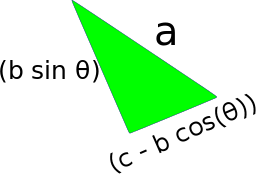### Site Tools

en:proof-of-the-law-of-cosines

# Proof of The Law of CosinesLaw of Cosines: a2 = b2 + c2 - 2bccos(θ)

## Proof

Divide the triangle into 2 right angle triangles:Using trigonometry, the sides of the green triangle are

• Longest side: a
• Side on the left: b . sin θ ; because the sine of θ is opposite divided the hypotenuse, and the opposite is the length of this side. Then, the length of this side is the hypotenuse multiplied by the sine of θ
• Side on the right: *c - b cos(θ) ; we must subtract from the length “c”, the part of c that is occupied by the red triangle. We know that, in the red triangle, cos θ is the adjacent side divided the hypotenuse. The adjacent is the “base” of the red triangle, and it is cos θ multiplied by the hypotenuse. The hypotenuse is b. Then, the base of the red triangle is (cos θ)b. So, the length of the “base” of the green triangle is c - b.cos θUsing the Pythagorean Theorem:

a2 = (b sin θ)2 + (c - b cos(θ))2

= b2 sin 2 θ + c2 - 2cbcos(θ) + b 2 cos 2 θ

= b2 (sin 2 θ + cos 2 θ) + c2 - 2cbcos(θ)

Knowing that (sin 2 θ + cos 2 θ = 1) 1)

= b2 * 1 + c2 - 2cbcos(θ)

a2 = b2 + c2 - 2cbcos(θ)

## Discussion

Enter your comment. Wiki syntax is allowed:
Y A﻿ U I W